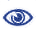# Prague Stringology Conference 2014

New Tabulation and Sparse Dynamic Programming Based Techniques for Sequence Similarity Problems

 Abstract: Calculating the length of a longest common subsequence (LCS) of two strings, A of length n and B of length m, is a classic research topic, with many worst-case oriented results known. We present two algorithms for LCS length calculation with respectively O(m n log log n / log2 n) and O(m n / log2 n + r) time complexity, the latter working for r = o(m n / (log n log log n)), where r is the number of matches in the dynamic programming matrix. We also describe conditions for a given problem sufficient to apply our techniques, with several concrete examples presented, namely the edit distance, LCTS and MerLCS problems.

 Download paper:PostScript PDF BibTeX reference
 Download presentation: